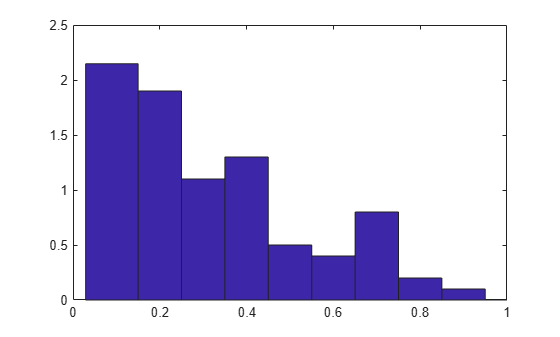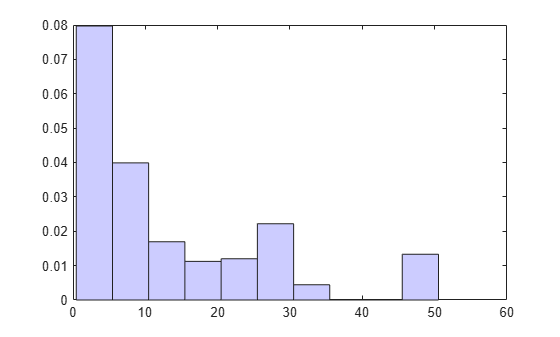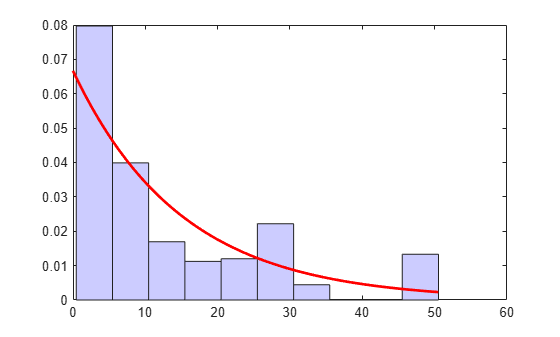Documentation

### This is machine translation

Mouseover text to see original. Click the button below to return to the English version of the page.

# ecdfhist

Histogram based on empirical cumulative distribution function

## Syntax

``````[n,c] = ecdfhist(f,x)``````
``````[n,c] = ecdfhist(f,x,m)``````
``n = ecdfhist(f,x,centers)``
``ecdfhist(___)``

## Description

example

``````[n,c] = ecdfhist(f,x)``` returns the heights, `n`, of histogram bars for 10 equally spaced bins and the position of the bin centers, `c`. `ecdfhist` computes the bar heights from the increases in the empirical cumulative distribution function, `f`, at evaluation points, `x`. It normalizes the bar heights so that the area of the histogram is equal to 1. In contrast, `histogram` produces bars with heights representing bin counts.```

example

``````[n,c] = ecdfhist(f,x,m)``` returns the histogram bars using `m` bins.```

example

````n = ecdfhist(f,x,centers)` returns the heights of the histogram bars with bin centers specified by `centers`.```

example

````ecdfhist(___)` plots the histogram bars.```

## Examples

collapse all

Compute the histogram bar heights based on the empirical cumulative distribution function.

Generate failure times from a Birnbaum-Saunders distribution.

```rng('default') % for reproducibility failuretime = random('birnbaumsaunders',0.3,1,100,1);```

Assuming that the end of the study is at time 0.9, mark the generated failure times that are larger than 0.9 as censored data and store that information in a vector.

```T = 0.9; cens = (failuretime>T);```

Compute the empirical cumulative distribution function for the data.

`[f,x] = ecdf(failuretime,'censoring',cens);`

Now, find the bar heights of the histogram using the cumulative distribution function estimate.

```[n,c] = ecdfhist(f,x); [n' c']```
```ans = 10×2 2.3529 0.0715 1.7647 0.1565 1.4117 0.2415 1.5294 0.3265 1.0588 0.4115 0.4706 0.4965 0.4706 0.5815 0.9412 0.6665 0.2353 0.7515 0.2353 0.8365 ```

Compute the bar heights for six bins using the empirical cumulative distribution function and also return the bin centers.

Generate failure times from a Birnbaum-Saunders distribution.

```rng('default') % for reproducibility failuretime = random('birnbaumsaunders',0.3,1,100,1);```

Assuming that the end of the study is at time 0.9, mark the generated failure times that are larger than 0.9 as censored data and store that information in a vector.

```T = 0.9; cens = (failuretime>T);```

First, compute the empirical cumulative distribution function for the data.

`[f,x] = ecdf(failuretime,'censoring',cens);`

Now, estimate the histogram with six bins using the cumulative distribution function estimate.

```[n,c] = ecdfhist(f,x,6); [n' c']```
```ans = 6×2 1.9764 0.0998 1.7647 0.2415 1.1294 0.3831 0.4235 0.5248 0.7764 0.6665 0.2118 0.8081 ```

Draw the histogram of the empirical cumulative distribution histogram for specified bin centers.

Generate failure times from a Birnbaum-Saunders distribution.

```rng default; % For reproducibility failuretime = random('birnbaumsaunders',0.3,1,100,1);```

Assuming that the end of the study is at time 0.9, mark the generated failure times that are larger than 0.9 as censored data and store that information in a vector.

```T = 0.9; cens = (failuretime>T);```

Define bin centers.

`centers = 0.1:0.1:1;`

Compute the empirical cumulative distribution function for the data and draw the histogram for specified bin centers.

```[f,x] = ecdf(failuretime,'censoring',cens); ecdfhist(f,x,centers) axis([0 1 0 2.5])```Generate right-censored survival data and compare the histogram from cumulative distribution function with the known probability distribution function.

Generate failure times from an exponential distribution with mean failure time of 15.

```rng default; % For reproducibility y = exprnd(15,75,1);```

Generate drop-out times from an exponential distribution with mean failure time of 30.

`d = exprnd(30,75,1);`

Record the minimum of these times as the observed failure times.

`t = min(y,d);`

Generate censoring by finding the generated failure times that are greater than the drop-out times.

`censored = (y>d);`

Calculate the empirical cdf and plot a histogram using the empirical cumulative distribution function.

```[f,x] = ecdf(t,'censoring',censored); ecdfhist(f,x) h = findobj(gca,'Type','patch'); h.FaceColor = [.8 .8 1]; hold on```Superimpose a plot of the known population pdf.

```xx = 0:.1:max(t); yy = exp(-xx/15)/15; plot(xx,yy,'r-','LineWidth',2) hold off```## Input Arguments

collapse all

Empirical cdf values at given evaluation points, `x`, specified as a vector.

For instance, you can use `ecdf` to obtain the empirical cdf values and enter them in `ecdfhist` as follows.

Example: `[f,x] = ecdf(failure); ecdfhist(f,x);`

Data Types: `single` | `double`

Evaluation points at which empirical cdf values, `f`, are calculated, specified as a vector.

For instance, you can use `ecdf` to obtain the empirical cdf values and enter them in `ecdfhist` as follows.

Example: `[f,x] = ecdf(failure); ecdfhist(f,x);`

Data Types: `single` | `double`

Number of bins, specified as a scalar.

For instance, you can draw a histogram with 8 bins as follows.

Example: `ecdfhist(f,x,8)`

Data Types: `single` | `double`

Center points of bins, specified as a vector.

Example: `centers = 2:2:10; ecdfhist(f,x,centers);`

Data Types: `single` | `double`

## Output Arguments

collapse all

Heights of histogram bars `ecdfhist` calculates based on the empirical cdf values, returned as a row vector.

Position of bin centers, returned as a row vector.